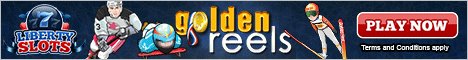# Sic Bo Odds and Bets

The outcome of sic bo bets are based on the combinations that arise from the roll of three dice. Winning sic bo bets can pay anywhere from 1:1 to 180:1. It is important to note that the odds may vary from casino to casino, and that the below sic bo odds are only representative of those found commonly at the Atlantic City casino and the Riviera. Most sic bo games do have these odds, however. Make sure to look into what the specifics are where you play, just to make sure. Below, you'll find a run down of these bets along with the odds associated with each bet. We will look at them in order from the bottom row of the table to the top row.

Single Number Bets
The bottom row of the table has six betting options. Here, you basically bet on what numbers will come up on each throw of the dice. If on the next roll one dice shows one of the numbers you bet on, you are paid at 1:1. If two numbers come up, you are paid at 2:1. If three come up, you guessed it, you're paid at 3:1. The probability of one number matching is 34.72%, for two matches it's 6.94%, and for three, it's 0.46%. The house edge on single number bets is 7.87%.

Two Number Combination Bets
The next row from the bottom contains fifteen betting options, known as combination bets. These are bets on any two dice combinations. For example, if you bet on two and six, and the next roll brings up a one, two and six, you win. However, it is only possible to win once on each roll, so if all three dice are numbers you picked (two of them being the same number), you will still only be paid once. These bets are paid at 6:1.

Three Number Total Bets
The next row, the third row from the bottom, contains fourteen betting options, known as the three number total bets. In these bets, you are betting on what the sum of the numbers will be of all three dice on the next roll. If the sum ends up being three or eighteen when you have made a wager on one of the three number total bets, you automatically lose. The following is a list of each three number total sic bo bet that includes what the probability of rolling it is, payouts and house edge:
- Four: Probability is 1.39%. Pays 50:1 or 60:1 (depending on casino). House edge is 29.17% or 15.28%.
- Five: Probability is 2.78%. Pays 18:1 or 30:1. House edge is 47.22% or 13.89%.
- Six: Probability is 4.63%. Pays 14:1 or 17:1. House edge is 30.56% or 16.67%.
- Seven: Probability is 6.94%. Pays 12:1. House edge is 9.72%.
- Eight: Probability is 9.72%. Pays 8:1. House edge is 12.50%.
- Nine: Probability is 11.57%. Pays 6:1. House edge is 18.98%.
- Ten: Probability is 12.50%. Pays 6:1. House edge is 12.50%.
- Eleven: Probability is 12.50%. Pays 6:1. House edge is 12.50%.
- Twelve: Probability is 11.57%. Pays 6 to 1 at both the Riviera and Atlantic City for house edge of 18.98%.
- Thirteen: Probability is 9.72%. Pays 8:1. House edge is 12.50%.
- Fourteen: Probability is 6.94%. Pays 12:1. House edge is 9.72%.
- Fifteen: Probability is 4.63%. Pays 14:1 or 17:1. House edge is 30.56% or 16.67%.
- Sixteen: Probability is 2.78%. Pays 18:1 or 30:1. House edge is 47.22% or 13.89%.
- Seventeen: Probability is 1.39%. Pays 50:1 or 60:1. House edge is 29.17% or 15.28%.

Triple and Pair Bets
The final, top row of the sic bo layout consists of five betting options (not including the Big and Small bets), and these bets are known as triple and pair bets. In addition to betting on only one type of triple, you can bet on all six possible triple combinations at once, which is known as Any Triplet. Additionally, you can bet on a pair, which is known as a pair bet. These are the specific bets:
- Double: This bet involves betting on a specific pair. You win if two (or three) of your chosen numbers appear. The probability of winning is 7.41% and it pays 8:1 or 10:1, with a house edge of 33.33% or 18.52%.
- Triple: This involves betting on any triple. You win if all dice are the number you chose. The probability of winning is 0.46%. It pays 150:1 or 180:1 and has a house edge of 30.09% or 16.20%.
- Two of a kind: Here, you bet on any of the 15 possible two dice combinations. You win if both numbers come up on the next roll. The probability of winning is 13.89% and it pays 5:1, with a house edge of 16.67%.
- Any Triple: This is a bet on any three of a kind. The probability of winning is 2.78%. It pays 24:1 or 30:1 and the house edge is 30.56% or 13.89%.

Small or Big Bets
Finally, also at the top row of the layout in the left and right corners, are the Big and Small bets. In these bets, you are betting on whether the sum of the next roll will be between four and ten (Small), or eleven and seventeen (Big). The probability of winning is 48.61% and it pays 1 to 1. The house edge is 2.78%. If the roll comes up as any triple, the bet loses.How to Play Sic Bo Sic Bo Layout Sic Bo Odds Sic Bo Strategy Download Free Sic Bo